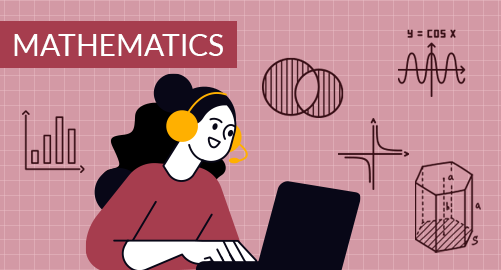#### Need Help?

Get in touch with usMathematics

Grade 10 Math covers Solving Equations and Inequalities, Linear equations and functions, Piecewise Functions, Exponential Functions, Polynomials and Factoring, Quadratic Functions and equations, Working with functions, Statistics, Polynomials and rational expressions

## What you'll learn

• 1

Solving Equations and Inequalities: Operations on Real Numbers, Solving Linear Equations, Solving Equations with a variable on both sides, Literal Equations and Formulas, Inequalities in one variable, Compound Inequalities, Absolute value Equations and Inequalities.

• 2

Linear equations and functions: Slope-Intercept form, Point slope form, Standard form, Parallel and perpendicular lines, Relations and functions, Linear functions, Transforming linear functions, Arithmetic sequences, Scatter plots and lines of fit, Analyzing lines of fit, Standard form, Parallel and perpendicular lines.

• 3

Piecewise Functions, Exponential Functions: The absolute value function, Piecewise-defined functions, Step functions, Transformations of piecewise-defined functions, Rational exponents and properties of exponents, Exponential functions, Exponential growth and decay, Modelling with geometric sequences, Transformations of exponential functions.

• 4

Polynomials and Factoring: Adding and subtracting polynomials, Multiplying polynomials, Multiplying special cases, Factoring polynomials, Factoring x2 + bx + c, Factoring special cases, Factoring ax2 + bx + c.

• 5

Quadratic Functions and equations: Key features of a quadratic function, Quadratic functions in vertex form, standard form, Modelling with quadratic functions, Linear, Exponential and quadratic models, Solving quadratic equations using graphs and tables, factoring, square roots, Rewriting radical expressions, Completing the square, The quadratic formula and the discriminant, Solving systems of linear and quadratic equations.

• 6

Working with functions: The square root function, The cube root function, Analyzing functions graphically, Translations of functions, Compressions and stretches of functions, Operations with functions, Inverse functions.

• 7

Statistics: Analyzing data displays, Comparing data sets, Interpret the shapes of data displays, Standard deviation, Two-way frequency tables, Data distributions, Normal distributions.

• 8

Polynomials and rational expressions: Polynomials identities, Dividing polynomials, Inverse variation and the reciprocal function, Multiplying and dividing rational expressions, Adding and subtracting rational expressions, Rational equations, Radical equations.

## Skills you'll learnIdentify an appropriate mathematical rule or procedure based on the classification of a given expressionExplain how an approximated value relates to the actual valueIdentify mathematical information from graphical, numerical, analytical, and/or verbal representationsDescribe the relationships among different. representations of functions and their derivativesExplain the meaning of mathematical solutions in contextIdentify an appropriate mathematical definition, theorem, or test to applyUse precise mathematical language and appropriate units of measure and symbols